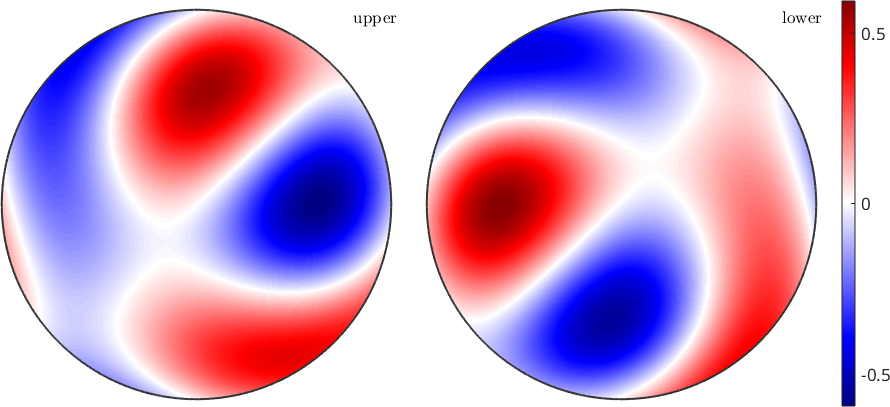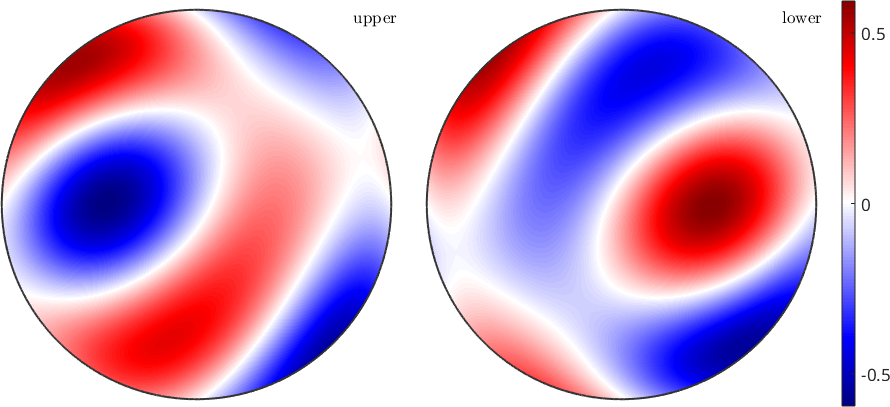The Piezoelectricity Tensor edit page

In this chapter we discuss how to compute and visualize piezoellectirc properties. At first, let us import some piezoelectric contents for a quartz specimen.

## Plotting the magnitude surface

The default plot of the magnitude, which indicates, in which direction we have the most polarization. By default, we restrict ourselves to the unique region implied by crystal symmetry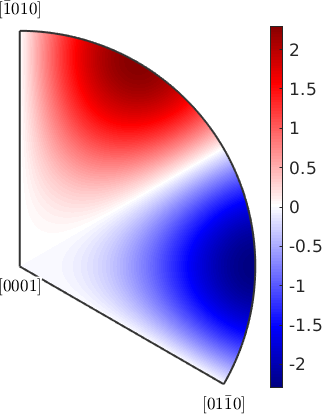but also, we can plot the whole crystal behavior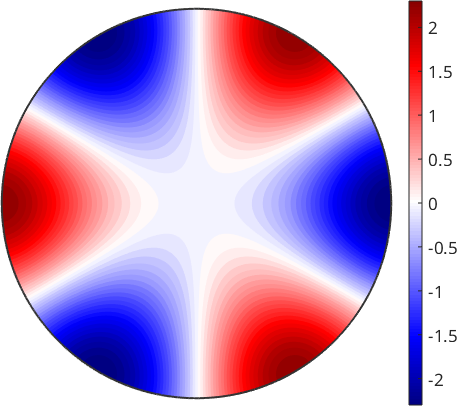Most often, the polarization is illustrated as surface magnitude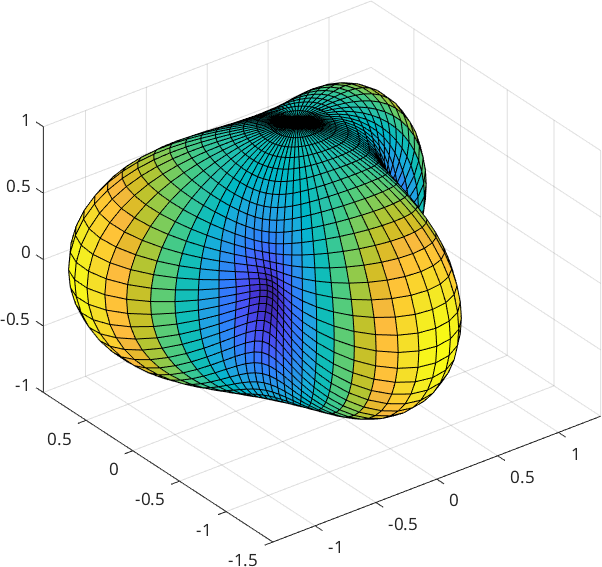Note, that for directions of negative polarization the surface is mapped onto the axis of positive, which then let the surface appear as a double coverage

Quite a famous example in various standard literature is a section through the surface because it can easily be described as an analytical solution. We just specify the plane normal vector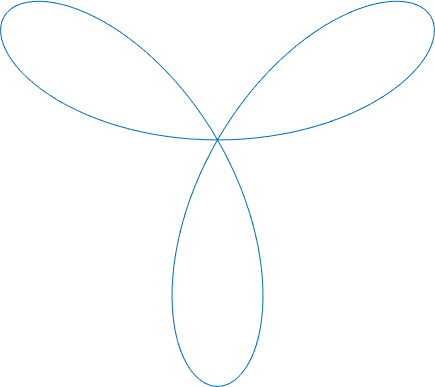so we are plotting the polarization in the xy-plane, or the yz-plane with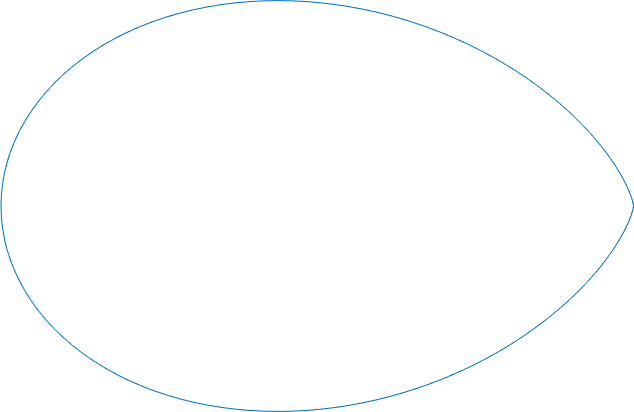## Mean Tensor Calculation

Let us import some data, which was originally published by Mainprice, D., Lloyd, G.E. and Casey , M. (1993) Individual orientation measurements in quartz polycrystals: advantages and limitations for texture and petrophysical property determinations. J. of Structural Geology, 15, pp.1169-1187

The figure on p.1184 of the publication